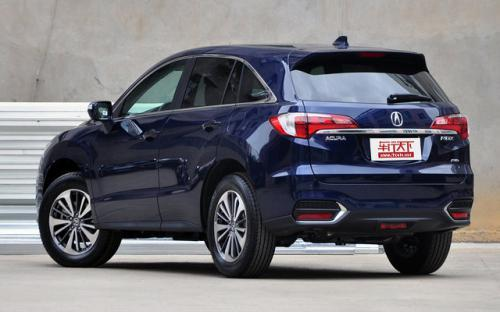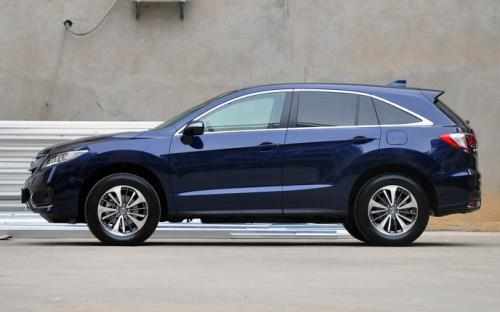讴歌（进口） 讴歌RDX6 种颜色可选2016款最低售价：39.98 万元起

4690(mm)1870(mm)1680(mm)配置亮点：
• 胎压监测装置

• ISOFIX儿童座椅接口

• 车身稳定控制(ESC/ESP/DSC等)

• 电动天窗

• 定速巡航

• 后倒车雷达

• 真皮座椅

• GPS导航系统

• 氙气大灯

• 后视镜加热

2016款 3.0L 两驱精锐版 (110张)
• 2016款 3.0L 两驱精锐版 (110张)
• 2016款 3.0L 四驱尊享版 (75张)讴歌（进口） 讴歌RDX 在售车型

排量 车型 厂商指导价 本地最低报价 购车工具
3.0L 3.0L 两驱舒适版 6挡手自一体 39.98万 39.98万

询底价+对比
3.0L 两驱精锐版 6挡手自一体 43.98万 43.98万

询底价+对比
3.0L 四驱尊享版 6挡手自一体 45.98万 45.98万

询底价+对比

讴歌（进口） 讴歌RDX 经销商

查看更多 >>
更多 >>

本地降价排名

讴歌（进口） 讴歌RDX 动力加速

讴歌RDX 0-100公里加速时间分布在 0.0-秒 属于 超跑级

动力级别 加速时间 车型

讴歌（进口） 讴歌RDX 视频

讴歌（进口） 讴歌RDX 新闻资讯

2015广州车展 讴歌新款RDX售价39.98万起

进口新车 超过9190次关注

广州车展上，讴歌正式推出了新款RDX，新车共3种配置，外观细节进行了小幅修改，内饰上配备了一块全新的液晶屏，取消了3.5L发动机，保留了3.0L发动机，官方指导售价...

搭9AT变速箱 讴歌新款RDX或四季度引入

进口新车 超过8464次关注

在近日开幕的2015上海车展上，我们从讴歌展台了解到，讴歌新款RDX或将在今年第四季度引入国内市场，新车在外观及内饰方面进行了一定的调整，并将匹配一款全新的9速...

讴歌RDX现身成都展馆将上市

车系文章 超过2875次关注

在成都车展开展前，我们在展馆发现了讴歌RDX的身影，这款车将于本次成都车展正式上市，预计售价在65万元左右。

试驾讴歌RDX 3.0L精英版 谁说我不在乎

车系文章 超过3806次关注

由于北京可恶的雷雨天气，在机场经过了近8个小时的等待，我拖着疲惫困倦的身体终于抵达了浙江舟山，来参加这次讴歌RDX两款车型（以下简称RDX）的试驾会，不过由于我...

讴歌RDX 3.0L车型6月8日上市

车系文章 超过1874次关注

日前，我们从讴歌官方获悉，讴歌将会在6月8日开幕的深港澳车展正式推出RDX的3.0L精英版车型。在今年除了RDX3.0L精英版外，讴歌ILX2.0L汽油版也将投放国内市场，届时...

预订即可提走现车 讴歌RDX少量现车销售

车系文章 超过4187次关注

近日，编辑从Acura郑州花园路店了解到，讴歌RDX店内有少量现车销售，近期价格比较稳定，暂无优惠，预订即可提走现车，感兴趣的朋友可致电4S店或直接到店赏车。

刚刚发布的海外小改款 官图解析讴歌RDX

车系文章 超过3747次关注

讴歌RDX对于国内消费者来说应该不会太熟悉，这是一款2006年讴歌推出的全新车型，定位于紧凑型SUV并将宝马X3视为自己的竞争对手。而随着奔驰GLK、沃尔沃XC60等车型的...

深港澳车展：讴歌RDX精英版售43.9万元

车系文章 超过4193次关注

2013年6月8日，讴歌在2013深港澳车展上正式推出RDX3.0L精英版车型，售价为43.9万元。此前，讴歌RDX在国内只有一款3.5L豪华版车型，而这一次推出3.0L精英版车型的主...

猜你喜欢

﻿
• 快速找车
• 选择品牌
• 选择品牌
• A  奥迪
• A  阿斯顿·马丁
• A  阿尔法·罗密欧
• B  宝沃
• B  布加迪
• B  巴博斯
• B  保时捷
• B  宾利
• B  奔驰
• B  宝马
• B  本田
• B  别克
• B  标致
• B  比亚迪
• B  宝骏
• B  北汽制造
• B  北汽新能源
• B  北汽幻速
• B  北汽威旺
• B  北京汽车
• B  奔腾
• B  北汽绅宝
• C  长安
• C  长安商用
• C  长城
• C  昌河
• D  大众
• D  道奇
• D  DS
• D  东南
• D  东风风神
• D  东风风行
• D  东风小康
• D  东风风度
• D  东风
• F  福特
• F  丰田
• F  菲亚特
• F  法拉利
• F  福田
• F  福迪
• F  福汽启腾
• G  观致
• G  广汽传祺
• G  广汽吉奥
• G  GMC
• H  红旗
• H  汉腾汽车
• H  哈弗
• H  哈飞
• H  海格
• H  海马
• H  华颂
• H  黄海
• H  华泰
• H  恒天
• J  吉利汽车
• J  捷豹
• J  Jeep
• J  江淮
• J  江铃
• J  金杯
• J  九龙
• J  金旅
• K  凯翼
• K  凯迪拉克
• K  克莱斯勒
• K  科尼塞克
• K  卡威
• K  开瑞
• L  路虎
• L  林肯
• L  劳斯莱斯
• L  兰博基尼
• L  雷克萨斯
• L  铃木
• L  雷诺
• L  理念
• L  力帆
• L  莲花汽车
• L  猎豹
• L  路特斯
• L  陆风
• M  马自达
• M  MG
• M  MINI
• M  玛莎拉蒂
• M  摩根
• M  迈凯轮
• N  纳智捷
• O  欧宝
• O  讴歌
• O  欧朗
• Q  奇瑞
• Q  起亚
• Q  启辰
• R  日产
• R  荣威
• R  瑞麒
• S  三菱
• S  斯威汽车
• S  萨博
• S  smart
• S  斯柯达
• S  斯巴鲁
• S  思铭
• S  双龙
• S  上汽大通
• S  双环
• T  特斯拉
• T  腾势
• W  沃尔沃
• W  五菱汽车
• W  五十铃
• W  威兹曼
• W  威麟
• X  现代
• X  雪佛兰
• X  雪铁龙
• X  西雅特
• Y  一汽
• Y  英菲尼迪
• Y  英致
• Y  依维柯
• Y  野马汽车
• Y  永源
• Z  众泰
• Z  中华
• Z  中兴
• Z  知豆
• 选择车系
• 选择车系
• 车型对比
• 选择品牌
• 选择品牌
• A  奥迪
• A  阿斯顿·马丁
• A  阿尔法·罗密欧
• B  宝沃
• B  布加迪
• B  巴博斯
• B  保时捷
• B  宾利
• B  奔驰
• B  宝马
• B  本田
• B  别克
• B  标致
• B  比亚迪
• B  宝骏
• B  北汽制造
• B  北汽新能源
• B  北汽幻速
• B  北汽威旺
• B  北京汽车
• B  奔腾
• B  北汽绅宝
• C  长安
• C  长安商用
• C  长城
• C  昌河
• D  大众
• D  道奇
• D  DS
• D  东南
• D  东风风神
• D  东风风行
• D  东风小康
• D  东风风度
• D  东风
• F  福特
• F  丰田
• F  菲亚特
• F  法拉利
• F  福田
• F  福迪
• F  福汽启腾
• G  观致
• G  广汽传祺
• G  广汽吉奥
• G  GMC
• H  红旗
• H  汉腾汽车
• H  哈弗
• H  哈飞
• H  海格
• H  海马
• H  华颂
• H  黄海
• H  华泰
• H  恒天
• J  吉利汽车
• J  捷豹
• J  Jeep
• J  江淮
• J  江铃
• J  金杯
• J  九龙
• J  金旅
• K  凯翼
• K  凯迪拉克
• K  克莱斯勒
• K  科尼塞克
• K  卡威
• K  开瑞
• L  路虎
• L  林肯
• L  劳斯莱斯
• L  兰博基尼
• L  雷克萨斯
• L  铃木
• L  雷诺
• L  理念
• L  力帆
• L  莲花汽车
• L  猎豹
• L  路特斯
• L  陆风
• M  马自达
• M  MG
• M  MINI
• M  玛莎拉蒂
• M  摩根
• M  迈凯轮
• N  纳智捷
• O  欧宝
• O  讴歌
• O  欧朗
• Q  奇瑞
• Q  起亚
• Q  启辰
• R  日产
• R  荣威
• R  瑞麒
• S  三菱
• S  斯威汽车
• S  萨博
• S  smart
• S  斯柯达
• S  斯巴鲁
• S  思铭
• S  双龙
• S  上汽大通
• S  双环
• T  特斯拉
• T  腾势
• W  沃尔沃
• W  五菱汽车
• W  五十铃
• W  威兹曼
• W  威麟
• X  现代
• X  雪佛兰
• X  雪铁龙
• X  西雅特
• Y  一汽
• Y  英菲尼迪
• Y  英致
• Y  依维柯
• Y  野马汽车
• Y  永源
• Z  众泰
• Z  中华
• Z  中兴
• Z  知豆
• 选择车系
• 选择车系
• 选择车型
• 选择车型
• 意见反馈